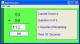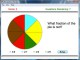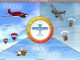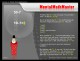Math Squared 1.0 Math Squared is a converter, calculator & practice program all in one. SharewareMaster Math Word Problems X 1.8.1 Master Math Word Problems is an easy to use program to aid students in learning to identify key words that identify mathematical operations and work through mathematical word problems. SharewareMath Helper 1.1 Math Helper is a math application that will let the user practice math operations while maintaining a log that records the results for progress tracking purposes. SharewareMaster Math Word Problems 1.2 Master Math Word Problems is an easy to use program to aide students in learning to identify key words that identify mathematical operations and work through mathematical word problems. SharewareMath Flash 4.6 Flash card style addition and subtraction practice. SharewareSnakey Math 2.2 Snakey Math is an educational game combining arithmetic practice with classic arcade action for one to four players. SharewareFRS Fraction Master 2.1 Learn fractions faster. SharewareMath Flight 2.3 Fly High while having fun learning basic mathematics with Math Flight! SharewareMath-Flight 2 2.3 Fly High while having fun learning basic mathematics with Math Flight! SharewareMental Math Master 1.2 Mental math skills in 3 minutes a day with this multimedia tutor. Demo